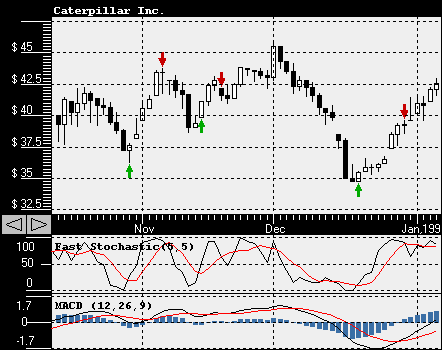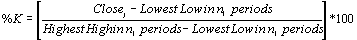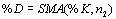# Fast Stochastic Oscillator

Fast Stochastic Oscillator is a momentum indicator that measures the price of a security relative to the high/low range over a fixed period of time. The indicator values range between 0 and 100, with readings below 20 considered oversold and readings above 80 considered overbought. A 5-period Fast Stochastic Oscillator reading of 30 would indicate that the current price was 30% above the lowest low of the last 5 days and 70% below the highest high. Fast Stochastic Oscillators was developed by George Lane in 1950s.These are two typical ways to generate stock signals using Fast Stochastic Oscillator: buy when %K (black line) or %D (red line) is below 20 and sell when %K or %D is rises above 80 level; or buy when %K is crossing %D in an upward direction and sell when %K is crossing %D in a downward direction.

Fast Stochastic Oscillator is calculated using the following formulas:here n1 and n2 are variable parameters and SMA is a simple moving average.

Stock Predictor - chart Fast Stochastic Oscillator and run trading strategies using it.